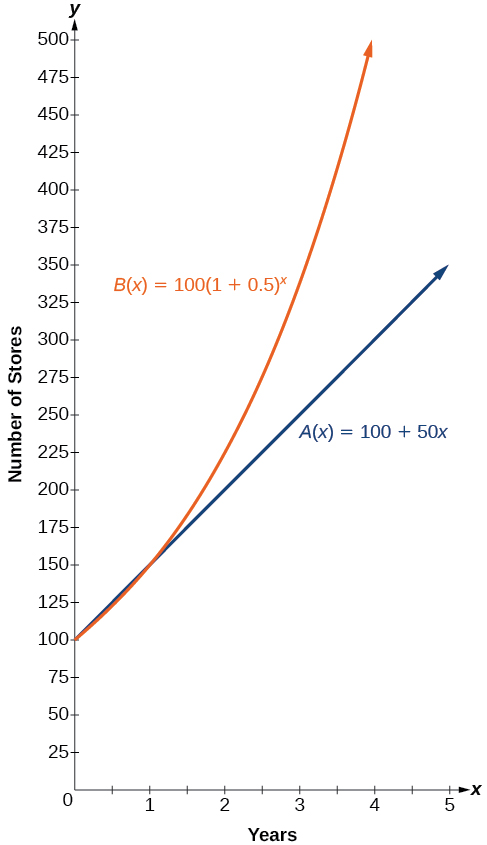# 6.1 Exponential functions  (Page 3/16)

 Page 3 / 16
• Let $\text{\hspace{0.17em}}b=1.\text{\hspace{0.17em}}$ Then $\text{\hspace{0.17em}}f\left(x\right)={1}^{x}=1\text{\hspace{0.17em}}$ for any value of $\text{\hspace{0.17em}}x.$

To evaluate an exponential function with the form $\text{\hspace{0.17em}}f\left(x\right)={b}^{x},$ we simply substitute $x\text{\hspace{0.17em}}$ with the given value, and calculate the resulting power. For example:

Let $\text{\hspace{0.17em}}f\left(x\right)={2}^{x}.\text{\hspace{0.17em}}$ What is $f\left(3\right)?$

To evaluate an exponential function with a form other than the basic form, it is important to follow the order of operations. For example:

Let $\text{\hspace{0.17em}}f\left(x\right)=30{\left(2\right)}^{x}.\text{\hspace{0.17em}}$ What is $\text{\hspace{0.17em}}f\left(3\right)?$

Note that if the order of operations were not followed, the result would be incorrect:

$f\left(3\right)=30{\left(2\right)}^{3}\ne {60}^{3}=216,000$

## Evaluating exponential functions

Let $\text{\hspace{0.17em}}f\left(x\right)=5{\left(3\right)}^{x+1}.\text{\hspace{0.17em}}$ Evaluate $\text{\hspace{0.17em}}f\left(2\right)\text{\hspace{0.17em}}$ without using a calculator.

Follow the order of operations. Be sure to pay attention to the parentheses.

Let $f\left(x\right)=8{\left(1.2\right)}^{x-5}.\text{\hspace{0.17em}}$ Evaluate $\text{\hspace{0.17em}}f\left(3\right)\text{\hspace{0.17em}}$ using a calculator. Round to four decimal places.

$5.5556$

## Defining exponential growth

Because the output of exponential functions increases very rapidly, the term “exponential growth” is often used in everyday language to describe anything that grows or increases rapidly. However, exponential growth can be defined more precisely in a mathematical sense. If the growth rate is proportional to the amount present, the function models exponential growth.

## Exponential growth

A function that models exponential growth    grows by a rate proportional to the amount present. For any real number $\text{\hspace{0.17em}}x\text{\hspace{0.17em}}$ and any positive real numbers and $\text{\hspace{0.17em}}b\text{\hspace{0.17em}}$ such that $\text{\hspace{0.17em}}b\ne 1,$ an exponential growth function has the form

where

• $a\text{\hspace{0.17em}}$ is the initial or starting value of the function.
• $b\text{\hspace{0.17em}}$ is the growth factor or growth multiplier per unit $\text{\hspace{0.17em}}x$ .

In more general terms, we have an exponential function , in which a constant base is raised to a variable exponent. To differentiate between linear and exponential functions, let’s consider two companies, A and B. Company A has 100 stores and expands by opening 50 new stores a year, so its growth can be represented by the function $\text{\hspace{0.17em}}A\left(x\right)=100+50x.\text{\hspace{0.17em}}$ Company B has 100 stores and expands by increasing the number of stores by 50% each year, so its growth can be represented by the function $\text{\hspace{0.17em}}B\left(x\right)=100{\left(1+0.5\right)}^{x}.$

A few years of growth for these companies are illustrated in [link] .

Year, $x$ Stores, Company A Stores, Company B
$0$ $100+50\left(0\right)=100$ $100{\left(1+0.5\right)}^{0}=100$
$1$ $100+50\left(1\right)=150$ $100{\left(1+0.5\right)}^{1}=150$
$2$ $100+50\left(2\right)=200$ $100{\left(1+0.5\right)}^{2}=225$
$3$ $100+50\left(3\right)=250$ $100{\left(1+0.5\right)}^{3}=337.5$
$x$ $A\left(x\right)=100+50x$ $B\left(x\right)=100{\left(1+0.5\right)}^{x}$

The graphs comparing the number of stores for each company over a five-year period are shown in [link] . We can see that, with exponential growth, the number of stores increases much more rapidly than with linear growth.The graph shows the numbers of stores Companies A and B opened over a five-year period.

Notice that the domain for both functions is $\text{\hspace{0.17em}}\left[0,\infty \right),$ and the range for both functions is $\text{\hspace{0.17em}}\left[100,\infty \right).\text{\hspace{0.17em}}$ After year 1, Company B always has more stores than Company A.

Now we will turn our attention to the function representing the number of stores for Company B, $\text{\hspace{0.17em}}B\left(x\right)=100{\left(1+0.5\right)}^{x}.\text{\hspace{0.17em}}$ In this exponential function, 100 represents the initial number of stores, 0.50 represents the growth rate, and $\text{\hspace{0.17em}}1+0.5=1.5\text{\hspace{0.17em}}$ represents the growth factor. Generalizing further, we can write this function as $\text{\hspace{0.17em}}B\left(x\right)=100{\left(1.5\right)}^{x},$ where 100 is the initial value, $\text{\hspace{0.17em}}1.5\text{\hspace{0.17em}}$ is called the base , and $\text{\hspace{0.17em}}x\text{\hspace{0.17em}}$ is called the exponent .

what are odd numbers
numbers that leave a remainder when divided by 2
Thorben
1,3,5,7,... 99,...867
Thorben
7%2=1, 679%2=1, 866245%2=1
Thorben
the third and the seventh terms of a G.P are 81 and 16, find the first and fifth terms.
if a=3, b =4 and c=5 find the six trigonometric value sin
pls how do I factorize x⁴+x³-7x²-x+6=0
in a function the input value is called
how do I test for values on the number line
if a=4 b=4 then a+b=
a+b+2ab
Kin
commulative principle
a+b= 4+4=8
Mimi
If a=4 and b=4 then we add the value of a and b i.e a+b=4+4=8.
Tariq
what are examples of natural number
an equation for the line that goes through the point (-1,12) and has a slope of 2,3
3y=-9x+25
Ishaq
show that the set of natural numberdoes not from agroup with addition or multiplication butit forms aseni group with respect toaaddition as well as multiplication
x^20+x^15+x^10+x^5/x^2+1
evaluate each algebraic expression. 2x+×_2 if ×=5
if the ratio of the root of ax+bx+c =0, show that (m+1)^2 ac =b^2m
By the definition, is such that 0!=1.why?
(1+cosA+IsinA)(1+cosB+isinB)/(cos@+isin@)(cos$+isin$)
hatdog
Mark
jaks
Ryan

#### Get Jobilize Job Search Mobile App in your pocket Now!ByByBy Dakota BocanBy Marion CabalfinBy OpenStaxBy Steve GibbsBy OpenStaxBy OpenStaxBy Brooke DelaneyBy Katy KeilersBy Courntey HubBy Brooke Delaney